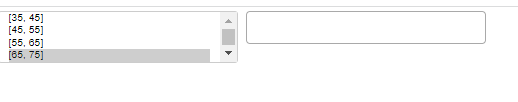# Multiselect option update params for sql query

Hi,

I am working on making a multiselect tool where the user selects a range, and that range is fed to a pd sql query. Can anyone point me to an example to help or maybe have a look at the code below (its only a snippet of what I am trying to achieve.

``````    OPTIONS = [("1", "[55, 65]"), ("2", "[65, 75]"), ("3", "[75, 85]"), ("4", "[85, 95]")]

multi_select = MultiSelect(value=[], options=OPTIONS)

multi_select.js_on_change("value", CustomJS(code="""
console.log('multi_select: value=' + this.value, this.toString())
"""))

db = _get_conn()
df1 = pd.read_sql_query(sql, db, params=[65.0, 75.0])

show(multi_select)

``````

the user selects a range, and that range is fed to a pd sql query.

Can you elaborate on what you want here? My guess is essentially you want the multiselect to allow the user to filter a dataset by multiple bin ranges?

Are you going the python–>bokeh server route or standalone–>CustomJS? Two drastically different options to implement depending on this…

Python → Bokeh Server.

Ill show a picture here:Image shows what I have today. User selects a range. That range is fed to the sql_query:

``````    db = _get_conn()
df1 = pd.read_sql_query(sql, db, params=[65, 75])
``````

I have added it manually here [65, 75]. The second multichoice changes based on the range the user inputs. I researched some more and I assume I need to use the on_change(‘value’, function). Where function is the database change request?

Yes that is correct.

You want to make a function that will:

1. Take the value selected by the user in the multiselect
2. Use that to run the pandas query, and
3. Then to use that query result to update the appropriate ColumnDataSource (aka the CDS that’s driving your graphs/whatever you have in bokeh.

And yes, apply that function to multiselect.on_change(‘value’,function). If you build up a dummy example here I can probably help further if you need it, but I think you’re on the right track.

1 Like

Heres a snippet of the solution I did to get it working:

``````from os.path import dirname, join
from bokeh.models import Div
import time
import pandas as pd
from app.db import _get_conn

from bokeh.io import show
from bokeh.layouts import column, row
from bokeh.models import CustomJS, MultiChoice, MultiSelect, Button

import json

sql = '''SELECT
test.temp
FROM test
WHERE test.temp BETWEEN %s AND %s
'''

OPTIONS = [
"[45, 55]",
"[55, 65]",
"[65, 75]",
"[75, 85]",
"[85, 95]"]

multi_select = MultiSelect(options=OPTIONS,
title = 'Select Temperature Range [deg C]:',
height = 200, width = 200)
multi_choice = MultiChoice(height = 150, width = 300, max_height = 170,
title = 'Search & Select:')
button = Button(label="Load", button_type="success")

def getField(value, sql):

db = _get_conn()
df = pd.read_sql_query(sql, db, params=value)

return df

def runSQLquery(attr, old, new):

print(attr, old, new)
df = getField(new, sql)

OPTIONS = list(df.Field.unique())
print(OPTIONS)
multi_choice.options = OPTIONS

def update_app(doc):

multi_select.on_change("value", runSQLquery)
multi_choice.js_on_change("value", CustomJS(code="""
console.log('multi_choice: value=' + this.value, this.toString())
"""))